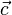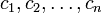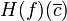# Difference between revisions of "Second derivative test for a function of multiple variables"

This article describes an analogue for functions of multiple variables of the following term/fact/notion for functions of one variable: second derivative test
This article describes a test that can be used to determine whether a point in the domain of a function gives a point of local, endpoint, or absolute (global) maximum or minimum of the function, and/or to narrow down the possibilities for points where such maxima or minima occur.
View a complete list of such tests

## Statement

Suppose$f$ is a function of a vector variable$\vec{x}$ with coordinates$x_1,x_2,\dots,x_n$, and suppose$\vec{c}$ is a point in the domain of$f$ with coordinates$c_1,c_2,\dots,c_n$. Suppose all the first-order partial derivatives of$f$ at$\vec{c}$ equal zero.

Suppose that all the second-order partial derivatives of$f$ at$\vec{c}$ (pure and mixed) exist and are continuous at and around$\vec{c}$. Note that Clairaut's theorem on equality of mixed partials thus applies and we get that$f_{x_ix_j}(\vec{c}) = f_{x_jx_i}(\vec{c})$. Also, continuous partials of a given order implies differentiable that many times tells us that$f$ is twice differentiable at$\vec{c}$. Combining all these pieces of information, we get that the Hessian matrix exists at$\vec{c}$ and is a symmetric matrix.

The second derivative test helps us determine whether$f$ has a local maximum at$\vec{c}$, local minimum at$\vec{c}$, or a saddle point at$\vec{c}$.

The test is as follows. We begin by computing the Hessian matrix of$f$ at$\vec{c}$. This is a$n \times n$ square matrix of real numbers. Further, due to Clairaut's theorem on equality of mixed partials, it is a symmetric matrix.

We can now state the test explicitly:

Condition on Hessian matrix How would we check this condition? Conclusion for$f$ at$\vec{c}$
The matrix is a positive definite matrix, i.e., the bilinear form induced by the matrix is positive definite. Since the matrix is a symmetric matrix, it suffices to check that all the principal minors have positive determinant. strict local minimum
The matrix is a negative definite matrix, i.e., the bilinear form induced by the matrix is negative definite. Check that the negative of the matrix is positive definite. Equivalently, the sign of the determinant of any principal minor is$(-1)^r$ where$r$ is the order of matrices. strict local maximum
The matrix is a positive semidefinite matrix but not a positive definite matrix. Since the matrix is a symmetric matrix, it suffices to check that all the principal minors have nonnegative determinant, but at least one of the determinants is zero. inconclusive. However, if any of the determinants for odd order minors is strictly positive, we can rule out the possibility of a maximum.
The matrix is a negative semidefinite matrix but not a negative definite matrix. Check that the negative of the matrix is positive semidefinite but not positive definite. inconclusive. However, if any of the determinants for odd order minors is strictly negative, we can rule out the possibility of a minimum.
The matrix is neither positive semidefinite nor negative semidefinite. Check that it satisfies none of the cases above. saddle point

## Facts used

1. Hessian matrix defines bilinear form that outputs second-order directional derivatives

## Proof

### Case of positive definite matrix

Given:$f$ is a function of a vector variable$\overline{x}$ with coordinates$x_1,x_2,\dots,x_n$, and suppose$\overline{c}$ is a point in the domain of$f$ with coordinates$c_1,c_2,\dots,c_n$. Suppose all the first-order partial derivatives of$f$ at$\overline{c}$ equal zero.

Suppose that all the second-order partial derivatives of$f$ at$\overline{c}$ (pure and mixed) exist and are continuous at and around$\overline{c}$. Note that Clairaut's theorem on equality of mixed partials thus applies and we get that$f_{x_ix_j}(\overline{c}) = f_{x_jx_i}(\overline{c})$. This forces the Hessian matrix$H(f)(\overline{c})$ to be symmetric.

Suppose further that the Hessian matrix$H(f)(\overline{c})$ is positive definite.

To prove:$f$ has a strict local minimum at$\overline{c}$.

Proof: The proof needs to be completed to address a subtlety.

Step no. Assertion/construction Facts used Given data used Previous steps used Explanation
1 For any unit vector$\overline{u}$, the second-order pure directional derivative $\overline{u}(D_{\overline{u}}(f))(\overline{c$ is positive. Fact (1) The Hessian matrix$H(f)(\overline{c})$ is positive definite -- Fact-Given combination direct
2 The restriction of$f$ to any straight line through$\overline{c}$ has a strict local minimum at$\overline{c}$. Moreover, ...